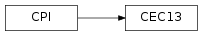# 5.3. `pyopus.problems.cec13` — Problems from the IEEE CEC 2013 competition¶CEC13 test problems (PyOPUS subsystem name: CEC13)

These are the test problems from the IEEE CEC 2013 real-parameter single objective optimization competition [cec13].

Objects of this class are callable. Calling them evaluates the corresponding function.

This module is independent of PyOPUS, meaning that it can be taken as is and used as a module in some other package. It depends only on the `cpi` and the `_cec13` modules.

The code in the binary module shares variables. If multiple CEC13 functions are created the initialization will take place automatically whenever a different function from the previous one is evaluated. Evaluation takes time so make sure you don’t switch functions frequently.

 [cec13] Liang J. J., Qu B. Y., Suganthan P. N., Hernández-Diaz A. G.: Problem Definitions and Evaluation Criteria for the CEC 2013 Special Session on Real-Parameter Optimization, Technical Report 201212, Computational Intelligence Laboratory, Zhengzhou University, Zhengzhou China, Technical Report, Nanyang Technological University, Singapore, 2013.
class `pyopus.problems.cec13.``CEC13`(name=None, number=None, n=10)

CEC13 test problems.

• name - problem name
• number - problem number (0-27)
• n - problem dimension

Attributes:

• `name` - problem name
• `n` - number of variables
• `xl` - vector of lower bounds on variables
• `xh` - vector of upper bounds on variables
• `xmin` - best known minimum position
• `fmin` - best known minimum value
`cpi`()

Returns the common problem interface.

Gradient is not supported.

See the `CPI` class for more information.

`names` = ['Sphere', 'RotatedElliptic', 'RotatedCigar', 'RotatedDiscus', 'DifferentPowers', 'RotatedRosenbrock', 'RotatedSchafferF7', 'RotatedAckley', 'RotatedWeierstrass', 'RotatedGriewank', 'Rastrigin', 'RotatedRastrigin', 'DiscontinuousRotatedRastrigin', 'Schwefel', 'RotatedSchwefel', 'RotatedKatsuura', 'LunacekBiRastrigin', 'RotatedLunacekBiRastrigin', 'ExpandedGriewankRosenbrock', 'ExpandedSchafferF6', 'Composited1', 'Composited2', 'Composited3', 'Composited4', 'Composited5', 'Composited6', 'Composited7', 'Composited8']

List of all function names

Example file cec13.py in folder demo/problems/

```# CEC13 problems test suite

from pyopus.problems.cec13 import *
import numpy as np

if __name__=='__main__':
print("CEC13 problems, n=10")
for ii in range(len(CEC13.names)):
prob=CEC13(number=ii, n=10)

f0=prob(np.zeros(10))
fmincalc=prob(prob.xmin)

print("%2d: %40s: f0=%14e fmincalc=%14e fmin=%14e" % (ii, prob.name, f0, fmincalc, prob.fmin))
print()

```